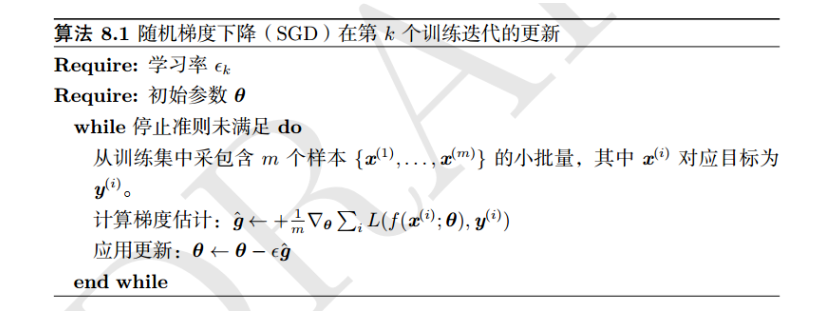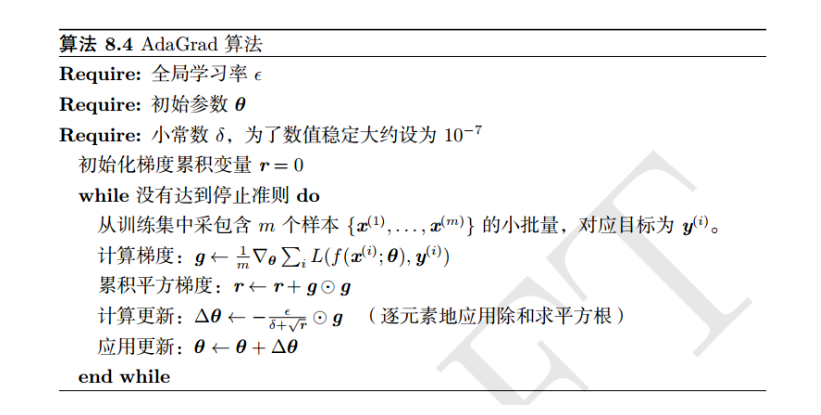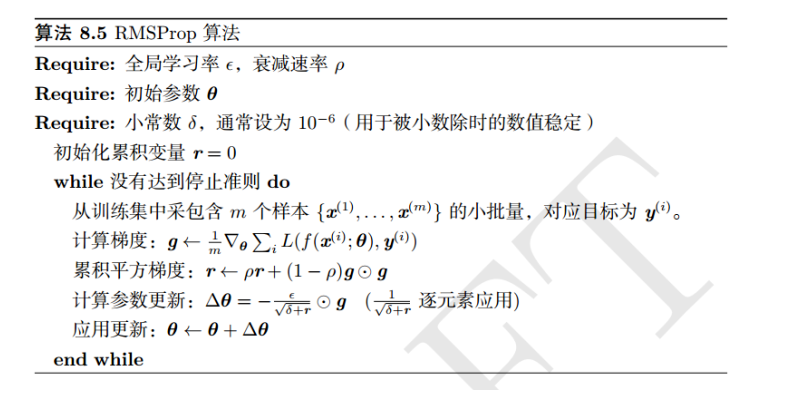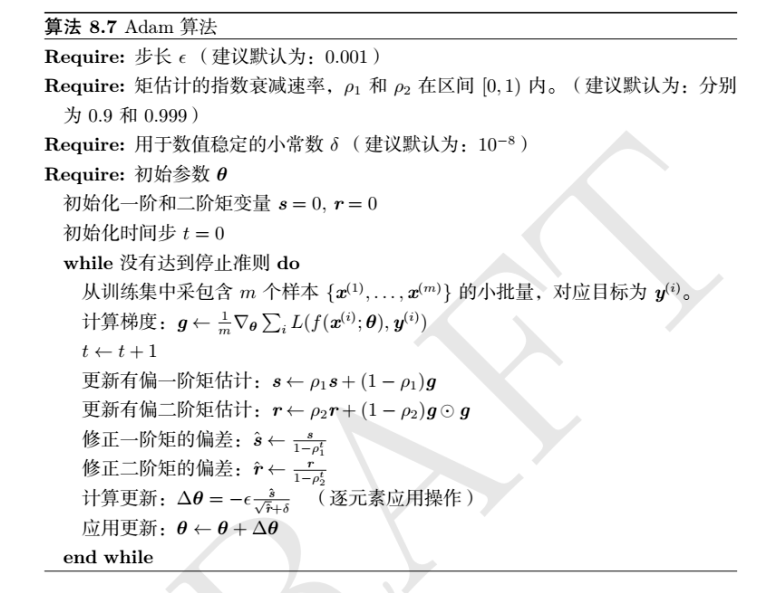• 在可接受的准确度下，高级算法可以减少训练时间(比如更少的迭代次数epochs)。
• 模型可以更好的适应其他超参数，而不仅仅是学习率。
• 理想情况下，可以获得比SGD更高的分类准确度。

## 自适应学习率

### Vanilla SGD

• W：模型权重矩阵
• lr:：学习率
• dW：W的梯度$n_{t} = v * n_{t-1} + (1- v) * g^2_{t}$

$\Delta \theta_{t} = - \frac{\eta}{\sqrt{n_{t} + \epsilon}} * g_{t}$

### RMSprop

$\gamma \leftarrow \rho \gamma + )1-\rho) g \odot g$m和v的值类似于SGD的momentum，依赖于t-1时刻之前的值，m表示梯度的第一时刻平均值，v表示梯度的第二时刻非中心方差值。• SGD
• RMSprop

## 总结

• 数据的熟悉
• 网络结构的熟悉
• 优化算法的熟悉(包括相关的超参数)